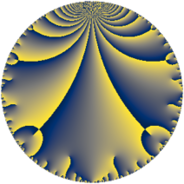# Properties

 Label 605.6.aLevel $605$ Weight $6$ Character orbit 605.a Rep. character $\chi_{605}(1,\cdot)$ Character field $\Q$ Dimension $181$ Newform subspaces $17$ Sturm bound $396$ Trace bound $2$

# Related objects

## Defining parameters

 Level: $$N$$ $$=$$ $$605 = 5 \cdot 11^{2}$$ Weight: $$k$$ $$=$$ $$6$$ Character orbit: $$[\chi]$$ $$=$$ 605.a (trivial) Character field: $$\Q$$ Newform subspaces: $$17$$ Sturm bound: $$396$$ Trace bound: $$2$$ Distinguishing $$T_p$$: $$2$$

## Dimensions

The following table gives the dimensions of various subspaces of $$M_{6}(\Gamma_0(605))$$.

Total New Old
Modular forms 342 181 161
Cusp forms 318 181 137
Eisenstein series 24 0 24

The following table gives the dimensions of the cuspidal new subspaces with specified eigenvalues for the Atkin-Lehner operators and the Fricke involution.

$$5$$$$11$$FrickeDim.
$$+$$$$+$$$$+$$$$42$$
$$+$$$$-$$$$-$$$$48$$
$$-$$$$+$$$$-$$$$48$$
$$-$$$$-$$$$+$$$$43$$
Plus space$$+$$$$85$$
Minus space$$-$$$$96$$

## Trace form

 $$181 q - 2 q^{2} + 40 q^{3} + 2896 q^{4} + 25 q^{5} - 24 q^{6} - 4 q^{7} - 732 q^{8} + 14265 q^{9} + O(q^{10})$$ $$181 q - 2 q^{2} + 40 q^{3} + 2896 q^{4} + 25 q^{5} - 24 q^{6} - 4 q^{7} - 732 q^{8} + 14265 q^{9} - 150 q^{10} + 2224 q^{12} + 14 q^{13} - 2484 q^{14} + 1000 q^{15} + 44724 q^{16} + 2634 q^{17} - 6618 q^{18} + 852 q^{19} + 3100 q^{20} + 640 q^{21} + 1088 q^{23} - 8584 q^{24} + 113125 q^{25} + 22436 q^{26} + 2320 q^{27} + 23556 q^{28} - 9594 q^{29} + 7800 q^{30} - 5468 q^{31} - 51256 q^{32} + 34056 q^{34} - 7900 q^{35} + 226264 q^{36} + 30142 q^{37} - 11580 q^{38} + 25248 q^{39} - 12600 q^{40} + 31666 q^{41} + 38924 q^{42} - 18928 q^{43} - 5675 q^{45} - 53336 q^{46} + 14956 q^{47} + 164940 q^{48} + 474889 q^{49} - 1250 q^{50} + 13536 q^{51} + 107836 q^{52} - 3286 q^{53} + 68456 q^{54} - 13328 q^{56} - 48160 q^{57} + 1076 q^{58} - 13976 q^{59} + 80900 q^{60} + 106230 q^{61} + 172672 q^{62} - 230740 q^{63} + 583052 q^{64} - 7250 q^{65} - 40004 q^{67} + 95748 q^{68} - 36648 q^{69} - 63200 q^{70} - 39828 q^{71} - 182316 q^{72} - 185134 q^{73} + 53204 q^{74} + 25000 q^{75} + 39728 q^{76} + 77212 q^{78} + 228688 q^{79} + 89600 q^{80} + 1028413 q^{81} - 300548 q^{82} - 345576 q^{83} + 514352 q^{84} - 88550 q^{85} - 21224 q^{86} - 24688 q^{87} - 268038 q^{89} + 50050 q^{90} + 418656 q^{91} - 222428 q^{92} + 953864 q^{93} - 193856 q^{94} - 117900 q^{95} + 711800 q^{96} + 280494 q^{97} + 436022 q^{98} + O(q^{100})$$

## Decomposition of $$S_{6}^{\mathrm{new}}(\Gamma_0(605))$$ into newform subspaces

Label Dim. $$A$$ Field CM Traces A-L signs $q$-expansion
$$a_2$$ $$a_3$$ $$a_5$$ $$a_7$$ 5 11
605.6.a.a $$1$$ $$97.032$$ $$\Q$$ None $$-2$$ $$-4$$ $$25$$ $$-192$$ $$-$$ $$-$$ $$q-2q^{2}-4q^{3}-28q^{4}+5^{2}q^{5}+8q^{6}+\cdots$$
605.6.a.b $$3$$ $$97.032$$ 3.3.21865.1 None $$7$$ $$-36$$ $$75$$ $$102$$ $$-$$ $$-$$ $$q+(2+\beta _{2})q^{2}+(-11-3\beta _{1})q^{3}+(12+\cdots)q^{4}+\cdots$$
605.6.a.c $$4$$ $$97.032$$ $$\mathbb{Q}[x]/(x^{4} - \cdots)$$ None $$5$$ $$0$$ $$-100$$ $$90$$ $$+$$ $$-$$ $$q+(1+\beta _{1})q^{2}+(\beta _{1}-\beta _{3})q^{3}+(15+\beta _{1}+\cdots)q^{4}+\cdots$$
605.6.a.d $$5$$ $$97.032$$ $$\mathbb{Q}[x]/(x^{5} - \cdots)$$ None $$-9$$ $$0$$ $$125$$ $$-70$$ $$-$$ $$-$$ $$q+(-2+\beta _{1})q^{2}+(\beta _{1}-\beta _{3})q^{3}+(24+\cdots)q^{4}+\cdots$$
605.6.a.e $$6$$ $$97.032$$ $$\mathbb{Q}[x]/(x^{6} - \cdots)$$ None $$-3$$ $$0$$ $$-150$$ $$66$$ $$+$$ $$-$$ $$q+(-1+\beta _{1})q^{2}+(-\beta _{1}-\beta _{3})q^{3}+(20+\cdots)q^{4}+\cdots$$
605.6.a.f $$7$$ $$97.032$$ $$\mathbb{Q}[x]/(x^{7} - \cdots)$$ None $$-1$$ $$-27$$ $$175$$ $$-133$$ $$-$$ $$-$$ $$q-\beta _{1}q^{2}+(-4-\beta _{3})q^{3}+(15+\beta _{2}+\cdots)q^{4}+\cdots$$
605.6.a.g $$7$$ $$97.032$$ $$\mathbb{Q}[x]/(x^{7} - \cdots)$$ None $$1$$ $$-27$$ $$175$$ $$133$$ $$-$$ $$-$$ $$q+\beta _{1}q^{2}+(-4-\beta _{3})q^{3}+(15+\beta _{2}+\cdots)q^{4}+\cdots$$
605.6.a.h $$8$$ $$97.032$$ $$\mathbb{Q}[x]/(x^{8} - \cdots)$$ None $$0$$ $$0$$ $$-200$$ $$0$$ $$+$$ $$+$$ $$q+\beta _{1}q^{2}-\beta _{2}q^{3}+(13+\beta _{4})q^{4}-5^{2}q^{5}+\cdots$$
605.6.a.i $$9$$ $$97.032$$ $$\mathbb{Q}[x]/(x^{9} - \cdots)$$ None $$-1$$ $$9$$ $$-225$$ $$71$$ $$+$$ $$-$$ $$q-\beta _{1}q^{2}+(1-\beta _{1}-\beta _{3})q^{3}+(14-\beta _{1}+\cdots)q^{4}+\cdots$$
605.6.a.j $$9$$ $$97.032$$ $$\mathbb{Q}[x]/(x^{9} - \cdots)$$ None $$1$$ $$9$$ $$-225$$ $$-71$$ $$+$$ $$-$$ $$q+\beta _{1}q^{2}+(1-\beta _{1}-\beta _{3})q^{3}+(14-\beta _{1}+\cdots)q^{4}+\cdots$$
605.6.a.k $$10$$ $$97.032$$ $$\mathbb{Q}[x]/(x^{10} - \cdots)$$ None $$0$$ $$36$$ $$250$$ $$0$$ $$-$$ $$+$$ $$q+\beta _{1}q^{2}+(4-\beta _{2})q^{3}+(3^{3}+\beta _{2}+\beta _{4}+\cdots)q^{4}+\cdots$$
605.6.a.l $$14$$ $$97.032$$ $$\mathbb{Q}[x]/(x^{14} - \cdots)$$ None $$0$$ $$-18$$ $$-350$$ $$0$$ $$+$$ $$+$$ $$q+\beta _{1}q^{2}+(-1-\beta _{2})q^{3}+(3+\beta _{4}+\beta _{5}+\cdots)q^{4}+\cdots$$
605.6.a.m $$18$$ $$97.032$$ $$\mathbb{Q}[x]/(x^{18} - \cdots)$$ None $$0$$ $$54$$ $$450$$ $$0$$ $$-$$ $$+$$ $$q+\beta _{1}q^{2}+(3-\beta _{3})q^{3}+(21+\beta _{2}-\beta _{3}+\cdots)q^{4}+\cdots$$
605.6.a.n $$20$$ $$97.032$$ $$\mathbb{Q}[x]/(x^{20} - \cdots)$$ None $$-17$$ $$22$$ $$500$$ $$-559$$ $$-$$ $$-$$ $$q+(-1+\beta _{1})q^{2}+(1-\beta _{3})q^{3}+(13-\beta _{1}+\cdots)q^{4}+\cdots$$
605.6.a.o $$20$$ $$97.032$$ $$\mathbb{Q}[x]/(x^{20} - \cdots)$$ None $$-1$$ $$0$$ $$-500$$ $$167$$ $$+$$ $$-$$ $$q-\beta _{1}q^{2}-\beta _{4}q^{3}+(20+\beta _{2})q^{4}-5^{2}q^{5}+\cdots$$
605.6.a.p $$20$$ $$97.032$$ $$\mathbb{Q}[x]/(x^{20} - \cdots)$$ None $$1$$ $$0$$ $$-500$$ $$-167$$ $$+$$ $$+$$ $$q+\beta _{1}q^{2}-\beta _{4}q^{3}+(20+\beta _{2})q^{4}-5^{2}q^{5}+\cdots$$
605.6.a.q $$20$$ $$97.032$$ $$\mathbb{Q}[x]/(x^{20} - \cdots)$$ None $$17$$ $$22$$ $$500$$ $$559$$ $$-$$ $$+$$ $$q+(1-\beta _{1})q^{2}+(1-\beta _{3})q^{3}+(13-\beta _{1}+\cdots)q^{4}+\cdots$$

## Decomposition of $$S_{6}^{\mathrm{old}}(\Gamma_0(605))$$ into lower level spaces

$$S_{6}^{\mathrm{old}}(\Gamma_0(605)) \cong$$ $$S_{6}^{\mathrm{new}}(\Gamma_0(5))$$$$^{\oplus 3}$$$$\oplus$$$$S_{6}^{\mathrm{new}}(\Gamma_0(11))$$$$^{\oplus 4}$$$$\oplus$$$$S_{6}^{\mathrm{new}}(\Gamma_0(55))$$$$^{\oplus 2}$$$$\oplus$$$$S_{6}^{\mathrm{new}}(\Gamma_0(121))$$$$^{\oplus 2}$$